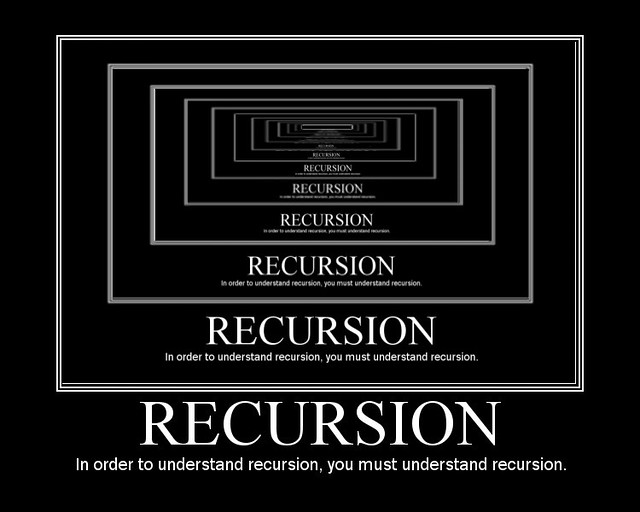Recursion in Elixir, We Don't Get No Loops!

in StemSociallast yearAs Elixir is a purely functional language, it doesn’t have loops. Instead, it uses a wide variety of recursive functions to achieve the same or better results.

Below is an example of a list and calculating it's length. This shows how recursive functions can be used to work like a loop.

Tail Call Optimization
A traditional function stack looks like this, with each member of the list becoming another function on the stack:

┌────────────────────────┐
│ length([1, 2, 3, 4], 0)│ <── Each member of the list
├────────────────────────┤ becomes a function on
│ length([2, 3, 4], 1)   │ the stack
├────────────────────────┤
│ length([3, 4], 2)      │
├────────────────────────┤
│ length(, 3)         │
├────────────────────────┤
│ length([], 4)          │
└────────────────────────┘

A tail call optimized function stack, like that used in Elixir, looks like this:

┌────────────────────────┐       ┌────────────────────────┐
│ length([1, 2, 3, 4], 0)│ <──── │ length([2, 3, 4], 1)   │
└────────────────────────┘       ├────────────────────────┤
│ length([3, 4], 2)      │
├────────────────────────┤
│ length(, 3)         │
├────────────────────────┤
│ length([], 4)          │
└────────────────────────┘

Function calls on the stack are replaced, so there is only one function on the stack at a time.

Requirements for Tail Call Optimization
In order to be tail call optimized, a function must call itself as the last operation. This is not a proper tail call:

def join([h|t]) do
h <> " " <> join(t)
end

But this is:

def join([h|t], string) do
string = string <> " " <> h
join(t, string)
end

So, now let's look at some examples of a list (MyList) and calling different recursive functions that allow us to work with the list's data.

MyList.length/1

defmodule MyList do
def length(list) do
length(list, 0)
end

defp length([], count) do
count
end

defp length([_|t], count) do
length(t, count + 1)
end
end

MyList.each/2

defmodule MyList do
def each([], _fun) do
:ok
end

def each([h|t], fun) do
fun.(h)
each(t, fun)
end
end

Usage:

MyList.each [1, 5, 12], fn(num) ->
IO.puts(num)
end
# 1
# 5
# 12
# => :ok

MyList.map/2

defmodule MyList do
def map(list, fun) do
do_map(list, fun, [])
end

defp do_map([], _fun, acc) do
:lists.reverse(acc)
end

defp do_map([h|t], fun, acc) do
result = fun.(h)
acc = [result | acc]
do_map(t, fun, acc)
end
end

The final do_map clause could also be shortened like this:

defp do_map([h|t], fun, acc) do
do_map(t, fun, [fun.(h) | acc])
end

Usage:

list = [
{"Nolan", 30},
{"Alex", 32}
]

MyList.map list, fn({name, _age}) ->
name
end

# => ["Nolan", "Alex"]